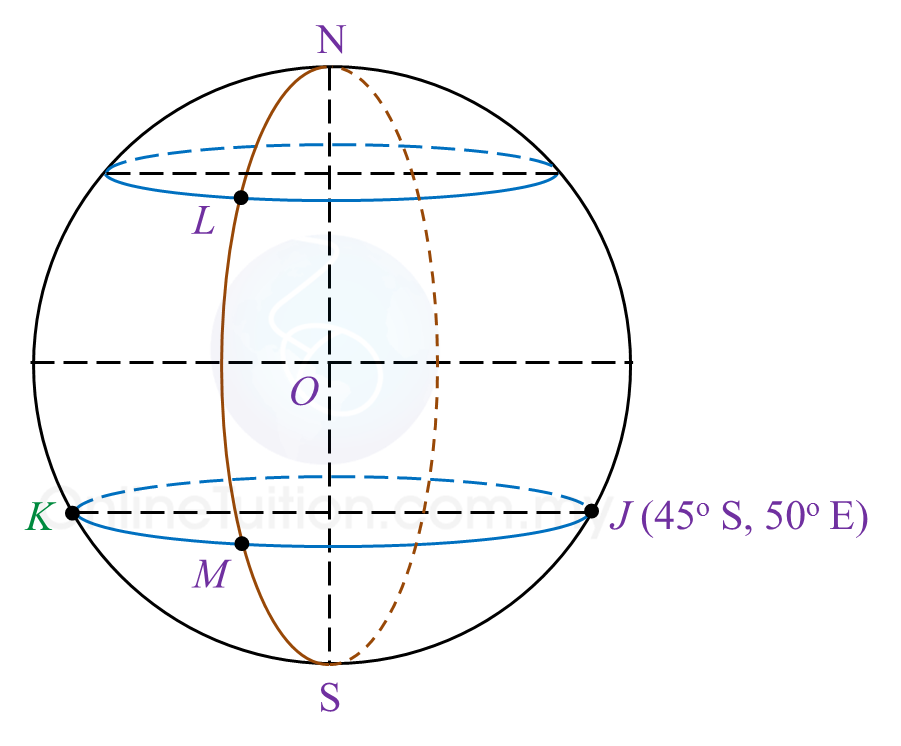# 9.6 SPM Practice (Long Questions)

Question 7 (12 marks):
Diagram 7 in the answer space shows the locations of points J, L and M, which lie on the surface of the earth. O is the centre of the earth. The longitude of M is 30o W. K is another point on the surface of the earth such that KJ is the diameter of the common parallel of latitude 45o S.

(a)(i)
Mark and label point K on Diagram 7 in the answer space.

(ii)
Hence, state the longitude of point K.

(b)
L lies due north of M and the shortest distance from M to L measured along the surface of the earth is 7500 nautical miles.
Calculate the latitude of L.

(c)
Calculate the distance, in nautical mile, from K due east to M measured along the common parallel of latitude.

(d)
An aeroplane took off from K and flew due east to M along the common parallel of latitude. The average speed of the aeroplane for the flight was 750 knots.
Calculate the total time, in hour, taken for the whole flight.Solution:
(a)(i)(a)(ii)
Longitude of point K = 130oW

(b)

(c)
KM = (130o – 30o) × 60 × cos 45o
= 4242.64 nautical miles

(d)